# SLAM Beginner's Program ~Input/Output~

## Introduction

I studied SLAM refer to the following book. I'm introducing my studying log in dividing into multiple articles. In this article, I wrote an system configuration of SLAM sample program.

## External sensor

• Distance measurement
One of the most important point on an external sensor is whether a distance can be measured or not.

• Measurement coverage
This is measurable minimum distance and maximum distance. Especially, if the maximum distance was narrow, a robot would not be able to sense many landmarks.

• Resolution
Resolution can be evaluated with the number of points per unit area. For example, it is the number of beam of laser scanner or an image resolution of camera.

• Field of View
A robot which has a large field of view can sense a good landmark quickly and can execute stable SLAM process.

• Illumination
A laser scanner and a camera can be used in a less lighting condition because they are an active sensor. On the other hand, in a extremely bright condition, almost laser scanner can be used but almost camera can not be used.
A dynamic range of the illumination is so large outside. The image of camera has a blown out highlights or a blocked up shadows. And then, a development of camera which can correspond a high dynamic range is desired.

• Feature value This is an information data which can be measured directly except a position and a direction. For example, it is a brightness or a color of camera, a reflection intensity of laser scanner.
In addition, neighbor points and feature value vector called local descriptor is calculated.

## Internal sensor

This sensor is used for estimating a state of robot without any external measurement. For example, odometry, gyroscope and IMU.
Wheel odometry calculates a velocity and yaw rate with a rotational frequency of wheel. And then, a robot position is calculated with the velocity and yaw rate. This method has an position error because of error accumulation or wheel slip.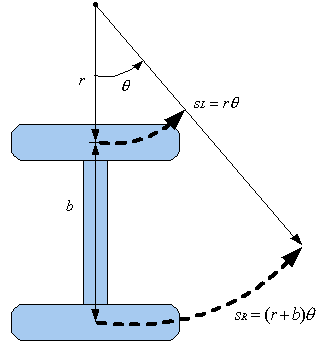Gyro scope can measure an rotation amount and yaw rate. 1-axis(yaw axis) gyro is used on 2D pose. 3-axis(roll, pitch and yaw) gyro is used on 3D pose. An accuracy of gyro is affected by temperature and include a accumulated error same as odometry.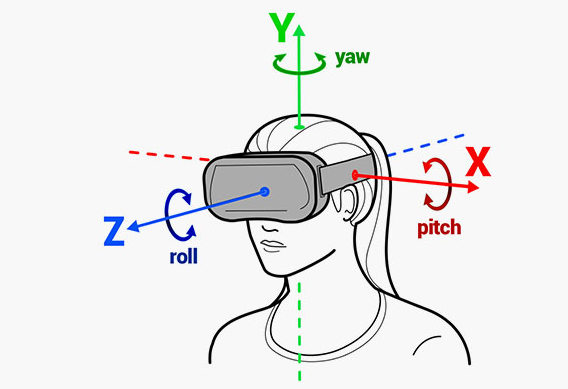IMU(Inertial Measurement Unit) includes gyro, acceleration sensor and magnetic sensor. The acceleration sensor is used for detecting an impact or controlling a pose of robot. The magnetic sensor is used for measuring a direction of magnetic and a direction of robot.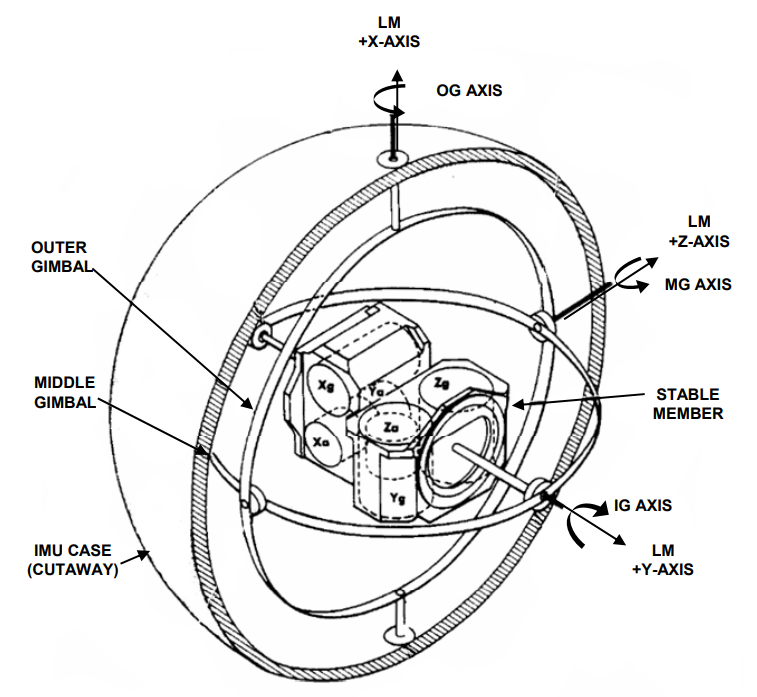## Robot pose

"Pose" includes both of position and direction. On robot coordinate system, x-y coordinate is defined on a ground surface where the robot moves. Moving direction of robot is defined as x axis and vertical direction is defined as z axis. To simplify this problem, a sensor pose and robot pose are defined as same.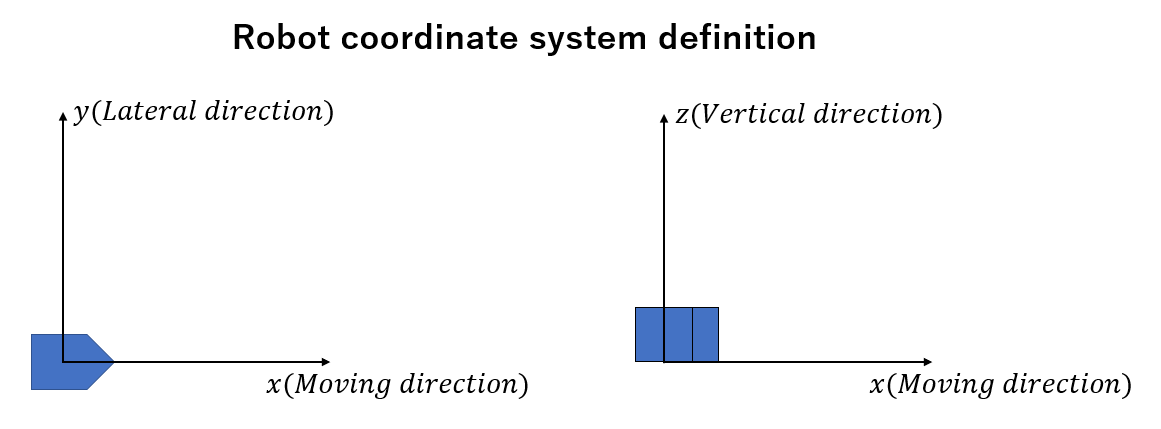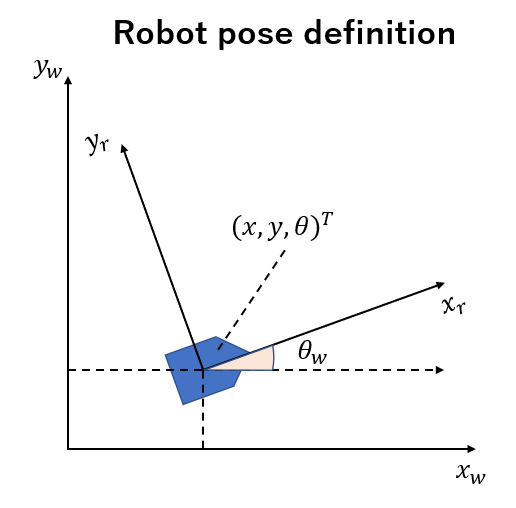## Map

There are various expression format of map data. Those format is as follow.

• Metrical map
• Topological map
• Feature map
• Occupancy grid map

### Metrical map

This map is which maintains a distance and a direction in the real world with a scale. Almost map in a map book have this format.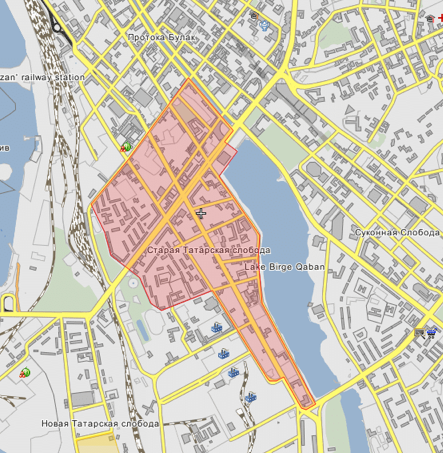### Topological map

This map maintains a connection of each location but does not maintain a correct distance and direction. This map does not have many environment information and the robot needs a powerful perception for navigation.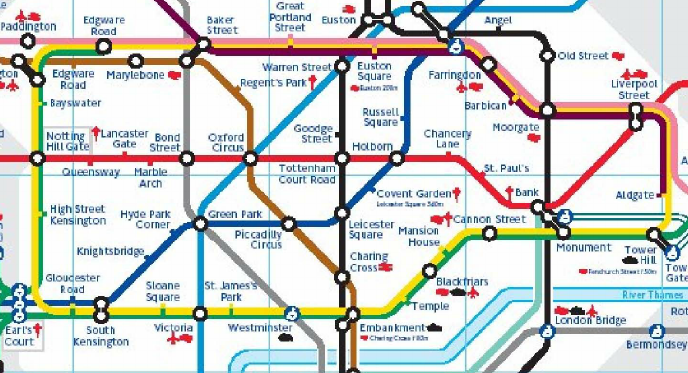### Feature map

A component of this map is a extracted feature from sensor data. The most basic feature extracted from sensor data is point. The following point cloud map with a lot of point data is commonly used.
Any other features except point are for example, straight line, line segment and plane. A higher degree feature is abstract and a lower degree feature has a strict constraint. SLAM commonly uses the point cloud map and detects the higher degree features for reducing data size and improving an abstraction.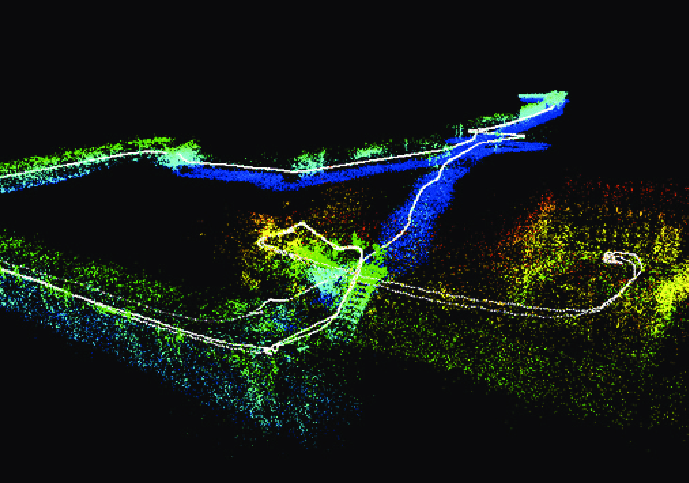### Occupancy grid map

Floor surface is split into multiple cells with grid at equal intervals. The occupancy grid map expresses a probability which each cell is occupied by an obstacle. Each cell have two types of state, occupied or free. And then, an occupied probability is used as a cell value.
In the following example, black is occupied probability 1, white is occupied probability 0 and gray is occupied probability 0.5. The occupied probability 0.5 expresses what the cell can not be judged as occupied or free.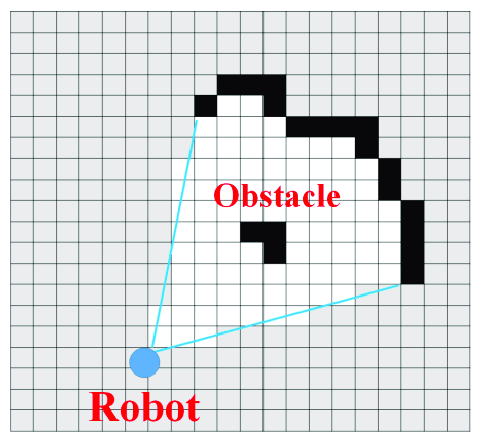#### Expressing change over time

The occupied probability is update every time sensor data is measured. So, when the measured object moved, the occupied probability of cell changes. Each cell should not keep all of past data and delete an old data for reflecting the change overtime immediately.

#### Disadvantage of Occupancy grid map

If the target environment was large, a used memory and process cost would increase. In addition, if a size of cell was small, the used memory would increase too because a resolution of space which can be expressed by the occupancy grid map depends on the cell size. And then, a grid map coordinate system is used in the grid expression. The coordinate transformation will be more complex than the point cloud expression.